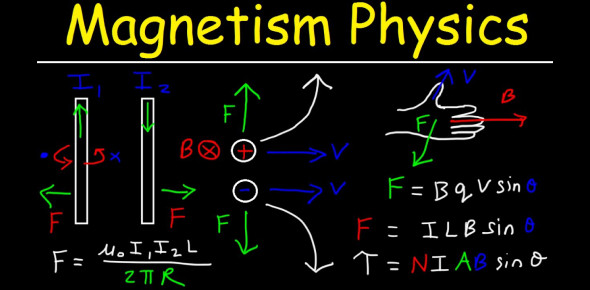# Physics Quiz: Theory Practice Questions On Magnetism!

13 Questions | Total Attempts: 616SettingsIn the scientific study of physics, magnetism is a class of phenomena which are mediated by magnetic fields. What can you tell us about this particular realm of science? Let’s take a look right now.

Related Topics
• 1.
Define magnet
• A.

A group of atoms with the magnetic fields lined up in the same direction

• B.

A material that attracts other magnets

• 2.
What are the two type of magnets
• A.

Alternating and direct

• B.

Strong and weak

• 3.
Like magnetic poles
• A.

Repel

• B.

Attract

• 4.
Describe a magnetic field
• A.

A material that attracts other magnets and causes the force that attracts or repels a magnet

• B.

Surrounds a magnet pointing from north pole to south pole and causes the force that attracts or repels a magnet

• 5.
Every magnetic field has poles: true or false
• A.

True

• B.

False

• 6.
An atom is:
• A.

The smallest magnet

• B.

A magnetic field

• C.

A magnetic domain

• 7.
A  magnetic domain :
• A.

Requires a group of atoms with the magnetic fields lined up in the same direction

• B.

Requires only a weak magnetic field to get domains to line up in the same direciton

• 8.
Strong magnetic material :
• A.

Is a group of atoms with the magnetic fields lined up in the same direction

• B.

Requires only a weak magnetic field to get domains to line up in the same direciton

• 9.
Earth is a magnet and has a magnetic field: true or false
• A.

True

• B.

False

• 10.
What causes earth magnetic field
• A.

Requires only a weak magnetic field to get domains to line up in the same direciton

• B.

A group of atoms with the magnetic fields lined up in the same direction

• C.

The motion of magma near the earths core

• 11.
How often does the earths magnetic field change directions
• A.

Every four to five thousand years

• B.

Every eight to ten years

• C.

Every four to five million years

• 12.
The earths magnetic field
• A.

Deflects charged particles from the sun and is used to determine direction by humans and animals

• B.

Requires only a weak magnetic field to get domains to line up in the same direciton

• C.

Is a group of atoms with the magnetic fields lined up in the same direction

• 13.
What is used to increase or decrease the voltage in ac system
• A.

Transformers

• B.

Voltmeter